# Plotting tools¶

Plastid includes a number of plotting tools built atop matplotlib:

Here, as elsewhere, we use the Demo dataset. Before starting, we download and unpack it, and also run a few scripts to produce files we’ll use below. From the terminal enter the following:

$wget https://www.dropbox.com/s/abktvrngn1lnzpb/plastid_demo.tar.bz2$ tar -jxvf plastid_demo.tar.bz2

# gene expression - for various scatter plots
$counts_in_region rp.txt --annotation_files merlin_orfs.gtf \ --count_files SRR609197_riboprofile_5hr_rep1.bam \ --fiveprime --offset 14$ counts_in_region mrna.txt --annotation_files merlin_orfs.gtf \
--count_files SRR592963_rnaseq_5hr_rep1.bam \
--center

# for metagene demo
$metagene generate merlin_start\ --upstream 50 --downstream 100 \ --annotation_files merlin_orfs.gtf$ metagene count merlin_start_rois.txt merlin_metagene \
--count_files SRR609197_riboprofile_5hr_rep1.bam \
--fiveprime --offset 14 --normalize_over 20 100 \
--keep


Then, in Python:

>>> import matplotlib.style
>>> matplotlib.style.use("ggplot")
>>> import matplotlib.pyplot as plt
>>>
>>> import numpy
>>> import pandas as pd
>>> from plastid import *
>>> from plastid.plotting.plots import *

# create some aliases for later
# we require positivity for log plots for convenience
>>> rpcounts = rp["counts"][rp["counts"] > 0]
>>> mcounts = mrna["counts"][rp["counts"] > 0]
>>> mcpn = mrna["counts_per_nucleotide"][mrna["counts_per_nucleotide"] > 0]
>>> lengths = rp["length"][rp["counts"] > 0]


## Kernel density plots¶

Kernel density plots are a continuous analog of histograms. These may be accessed via kde_plot().

# create some data
>>> a = numpy.hstack([25 + 5*numpy.random.randn(100),
>>>                   4 + 25*numpy.random.randn(50),
>>>                   -20 + numpy.random.randn(60)
>>>                  ])
>>> b = -5 + 2.5*numpy.random.randn(300)


Most of the plotting functions return a matplotlib Figure and a matplotlib Axes. To plot multiple data series on the same axes, pass the returned Axes instance back back to the axes parameter the second time you call the plotting function.

# plot kernel density estimates of A and B on the same axis
>>> fig, ax = kde_plot(a,label="series A")
>>> _,_ = kde_plot(b,axes=ax,label="series B")

# make things look nice
>>> plt.legend()
>>> plt.xlabel("Some unit")
>>> plt.ylabel("Probability density")
>>> plt.title("kde_plot() demo",y=1.1)


This produces the following: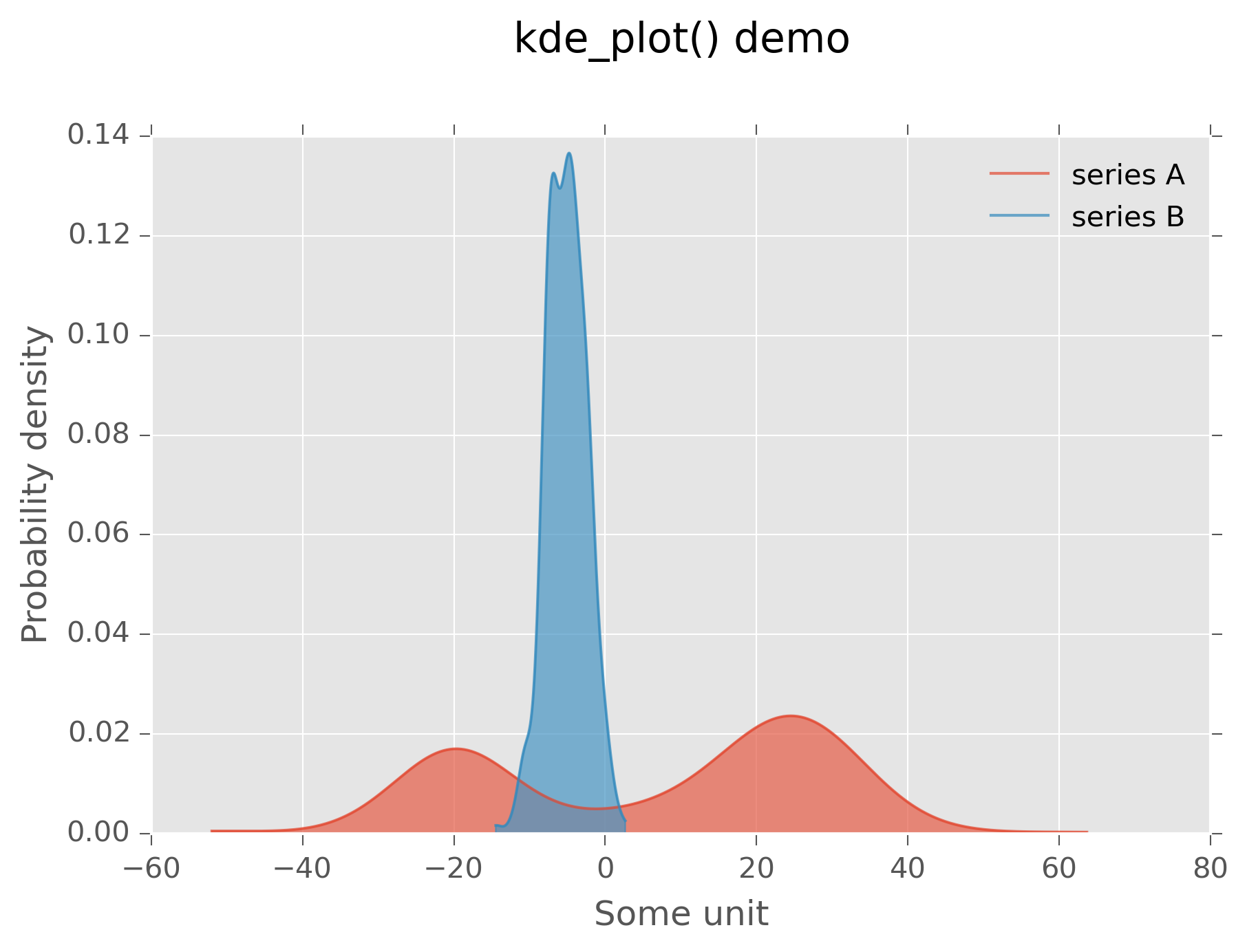Graphical output of plot above

One subtlety of kernel density estimates occurs when plotting in log space. In this case, kernel widths need to be scaled accordingly. This may be controlled via the log and base arguments:

>>> fig, ax = kde_plot(rpcounts,log=True,base=10,label="RP")
>>> _,_ = kde_plot(mcpn,axes=ax,log=True,base=10,label="mRNA")

>>> plt.xlabel("Counts or counts per nucleotide")
>>> plt.ylabel("Probability density")
>>> plt.legend(loc="upper right")
>>> plt.title("kde_plot() log demo",y=1.1)


This produces: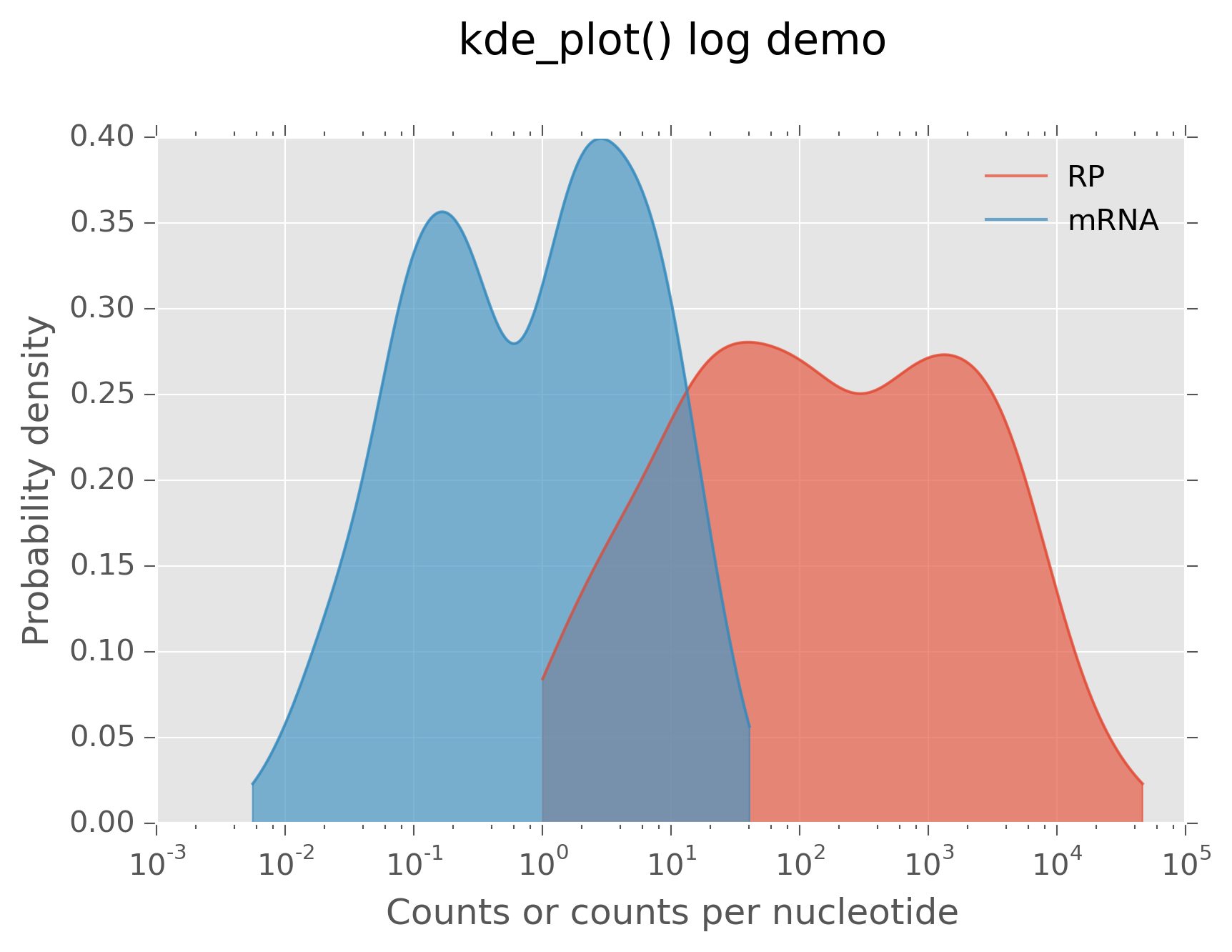Kernel density estimate in log space

## Scatter plots with marginal distributions¶

One use case for kernel density estimates is for better visualizing scatter plots. These may be made in plastid via scatterhist_x(), scatterhist_y(), and scatterhist_xy(), which plot the marginal distributions of points on the x, y, or x and y axes, respectively:

# generate some pseudo lengths - we'll pretend we have different genes
>>> fakelengths = numpy.random.randint(20,high=len(lengths),size=len(lengths))

>>> fig, ax = scatterhist_xy(lengths,mcounts,label="mRNA counts",
log="xy",min_x=0.1,min_y=0.1)

>>> _, _ = scatterhist_xy(fakelengths,rpcounts,label="RP counts",
log="xy",axes=ax,min_x=0.1,min_y=0.1)


Because these plots have multiple panes, instead of returning a single Axes, a dictionary of these is returned:

>>> mainax = ax["main"]
>>> mainax.legend(loc="lower right",frameon=True)
>>> mainax.set_xlabel("Length (nt)")

>>> ax["top"].yaxis.set_ticks([])
>>> ax["right"].xaxis.set_ticks([])
>>> ax["top"].set_title("scyatterhist_xy() demo",y=1.8)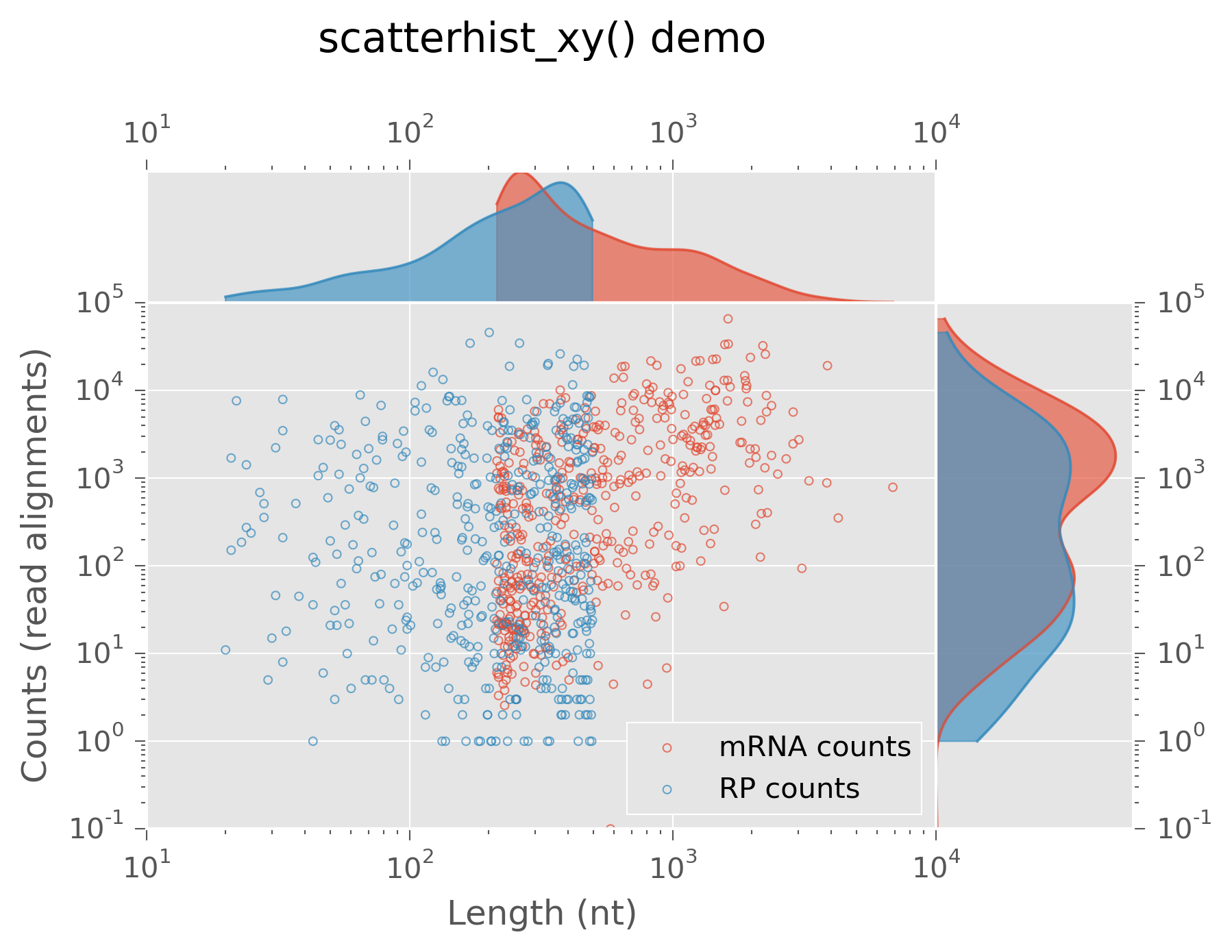Scatter plot with marginal densities

## MA plots¶

A specialized scatter plot is an MA plot, in which log2 ratios of two data series are plotted against their average. This is often used to visualize consistency between experimental replicates, or differential gene expression between different samples in RNA-seq or other sequencing experiments:

>>> fig, axes = ma_plot(rpcounts,mcounts)
>>> axes["main"].set_title("ma_plot() demo",y=1.1)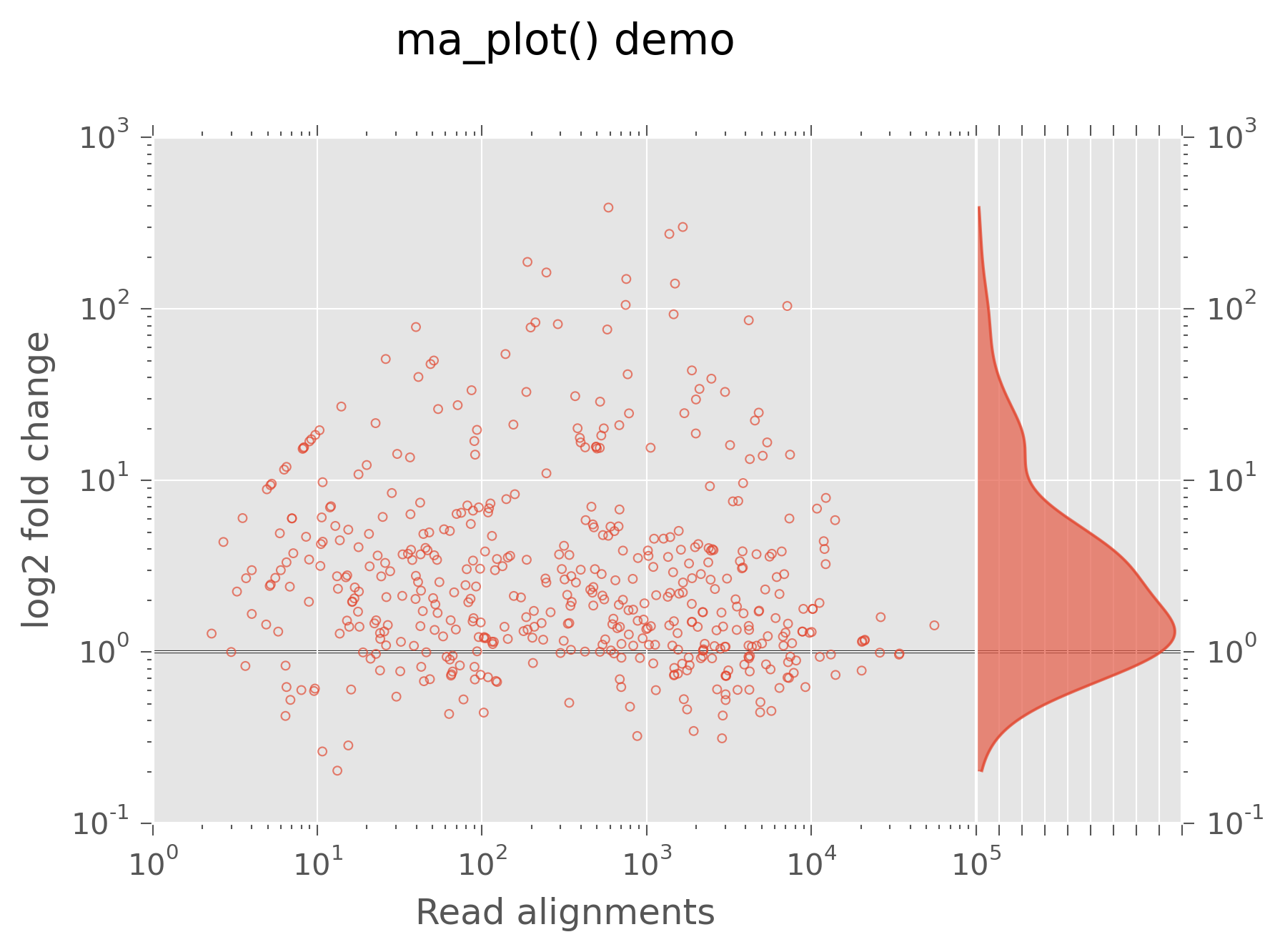MA plot, a scatter plot with marginal densities

## Stacked bar graphs¶

Stacked bar graphs may be created by providing a numpy.ndarray of data, in which each row becomes its own stacked bar. So, a 10x3 array would have 10 stacks, with 3 bars in each stack. Labels may be passed as a list to the labels argument. If a cmap is provided, colors for each sample will be generated as well:

>>> data = numpy.random.randint(0,high=100,size=(10,3),)
>>> fig, ax = stacked_bar(data,labels=list("abcdefhijk"),
cmap=matplotlib.cm.rainbow,lighten_by=0.3)

>>> plt.xlabel("Samples")
>>> plt.ylabel("Some quantity")
>>> plt.title("stacked_bar() demo",y=1.1)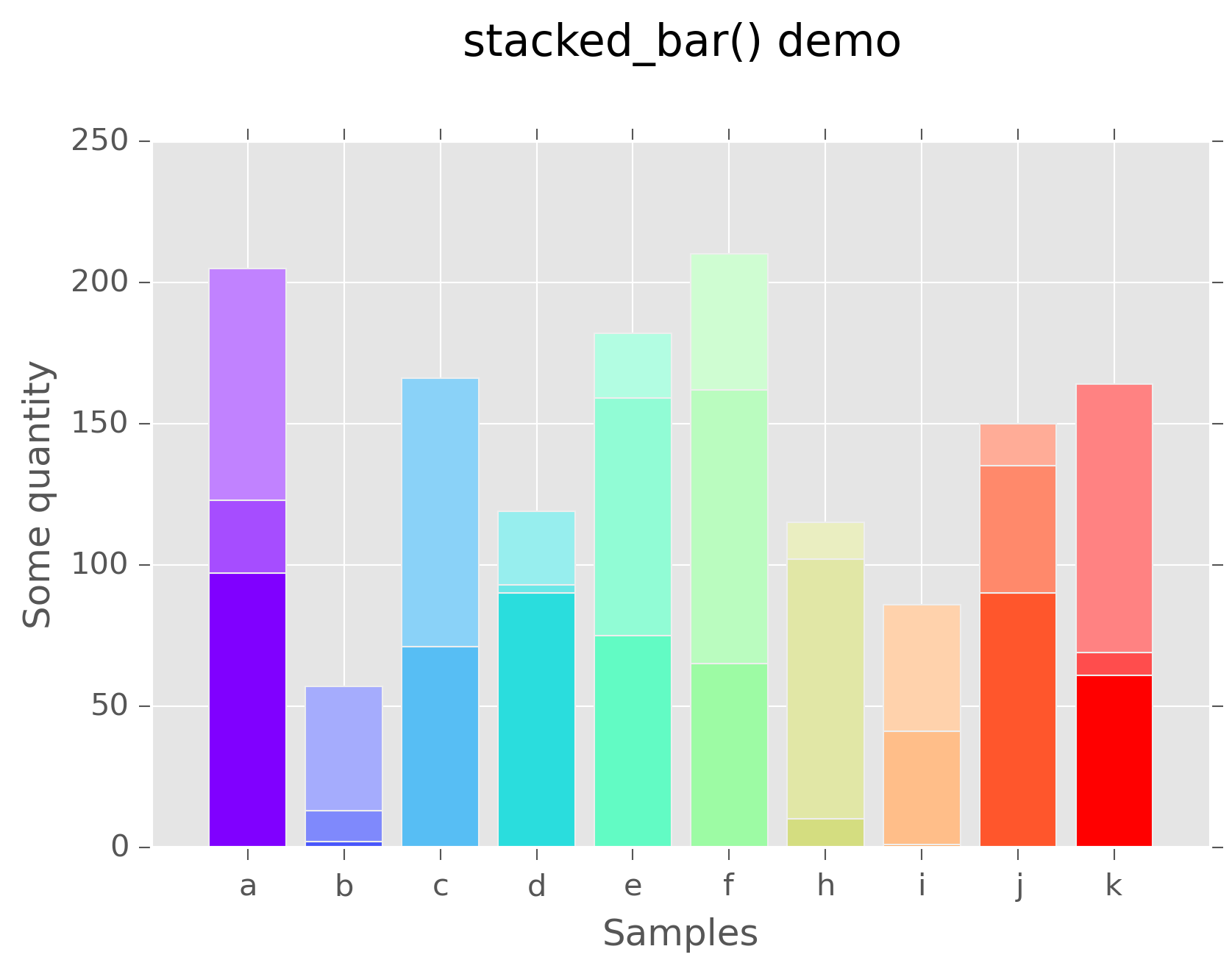Stacked bar graph

## Heatmaps with summary profiles (metagene plots)¶

When viewing a metagene average, it is often useful to look at the individual profiles underlying that average. This is the function of profile_heatmap(). As input, it takes a row-normalized matrix of counts, in which each row is a sample. If not provided as an optional argument, the profile, shown in the top panel, is made by taking the columnwise median of the data matrix. To make a plot:

# load raw and normalized count output from metagene

# exclude rows with few raw counts
>>> sums = (rc.sum(1) > 15)

# this dataset has extreme values, so we create a color normalizer
# to logscale colors, making them easier to see across the whole
# range of values
>>> norm = matplotlib.colors.SymLogNorm(0.0125,vmin=nc.min(),
>>>                                     vmax=nc.max(),clip=True)

>>> fig, ax = profile_heatmap(nc[sums],#numpy.log(0.01+nc[sums]),
>>>                           x=numpy.arange(-50,100),
>>>                           cmap=matplotlib.cm.Blues,
>>>                           im_args=dict(norm=norm))

# set titles and labels on specific axes
>>> ax["main"].set_xlabel("Distance from start codon (nt)")
>>> ax["main"].set_ylabel("Row-normalized ribosome density")
>>> ax["top"].set_title("Ribosome density surrounding start codons - Merlin data",y=1.8)


This yields: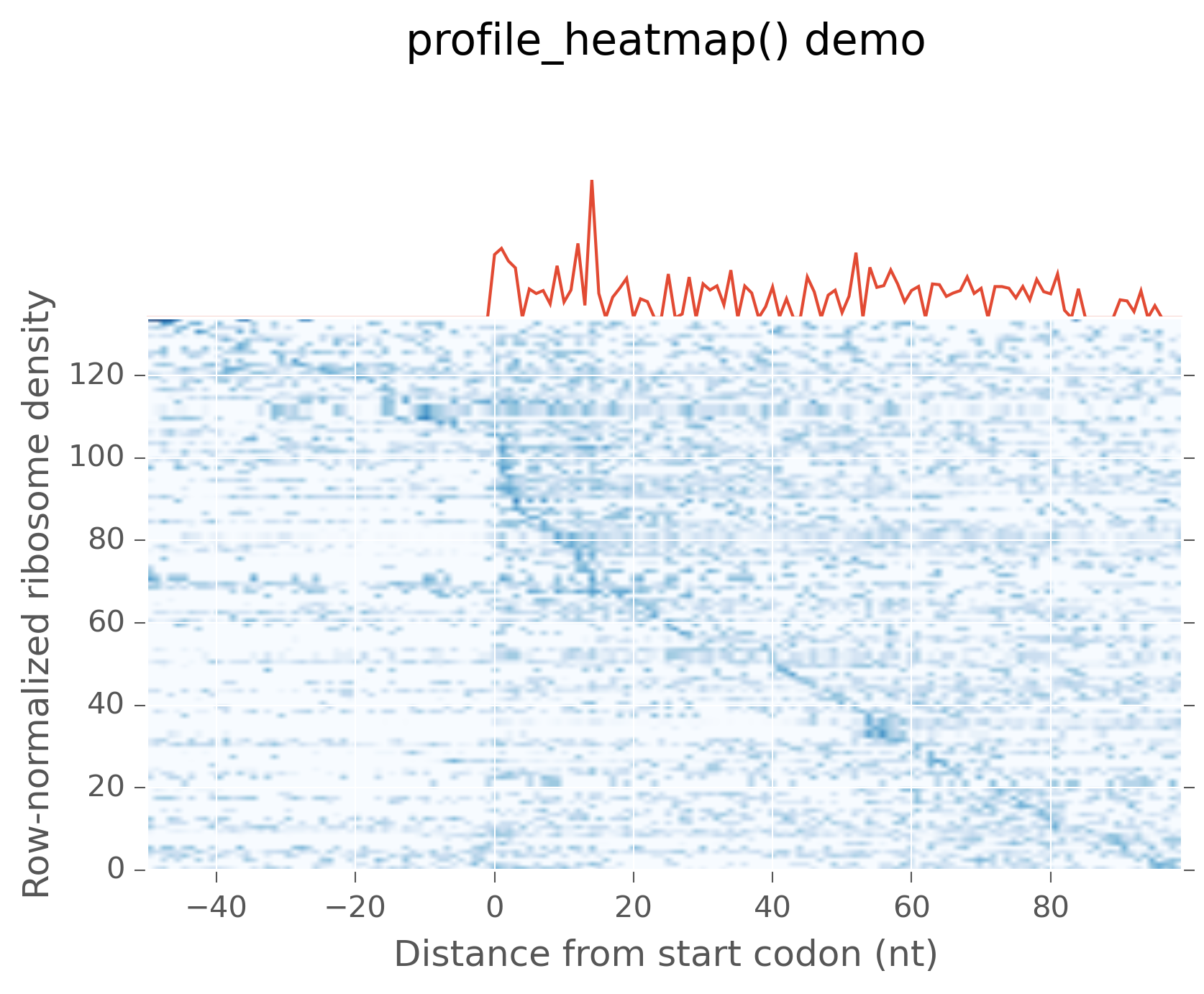Metagene average (top) with heatmap of individual profiles (bottom)

## Triangle plots¶

This is an unusual sort of plot, that can be used to visualize phasing. It is a homogeneous projection of the triangle defined by $$x + y + z \leq 1$$. In the case of ribosome profiling, x, y, and z would correspond to the fraction of ribosome-protected footprints appearing in each codon position.

# create some random data
>>> tripoints = numpy.random.randint(0,60,size=(25,3)).astype(float)
>>> tripoints[:,0] += numpy.random.randint(0,180,size=25)

# row-normalize it, each row adding to 1.0
>>> tripoints = (tripoints.T/tripoints.sum(1)).T

# let's give each point its own color
>>> cmap = matplotlib.cm.rainbow
>>> colors = cmap(numpy.linspace(0,1,25))


By default, data is plotted as a scatter plot, so we can pass keyword argments that are valid in scatter():

>>> fig, ax = triangle_plot(tripoints,grid=[0.5,0.75],
>>>                         marker="o",
>>>                         s=numpy.random.randint(40,400,size=25),
>>>                         linewidth=2,
>>>                         vertex_labels=["A","B","C"],
>>>                         edgecolor=colors,facecolor="none")


But, we can use most any matplotlib plotting functions that ordinarily takes a series of x points and a series of y points to draw the data in triangular space. We do this by passing the name of the method to the fn argument. For example, to draw a line using plot():

# plot a line using fn="plot", then pass appropriate keywords
>>> triangle_plot(tripoints,grid=[0.5,0.75],axes=ax,linewidth=0.5,
>>>               fn="plot",color="#222222",zorder=-2)

# give a title
>>> plt.title("triangle_plot() demo")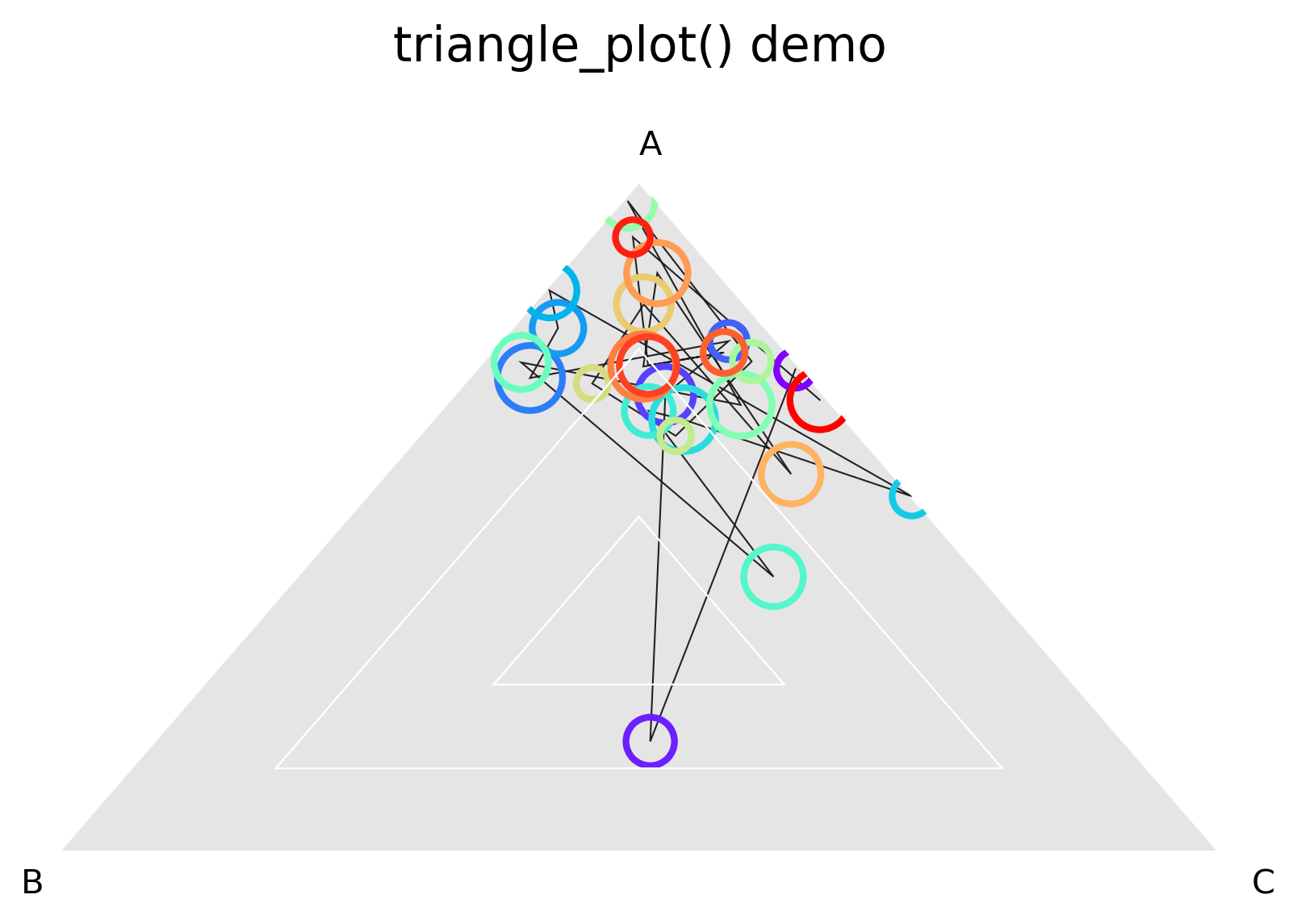Distance to each vertex indicates the magnitude of that column or phase